Publicité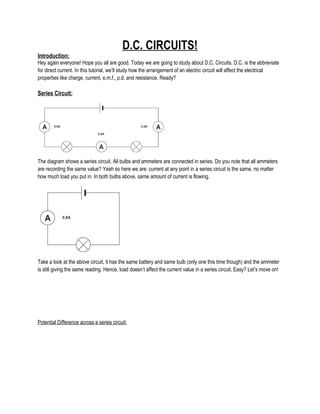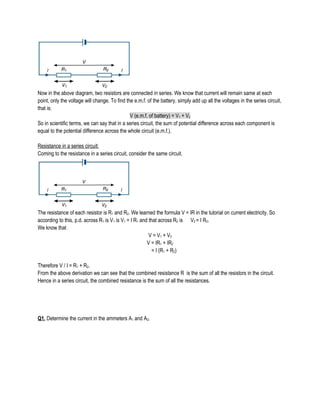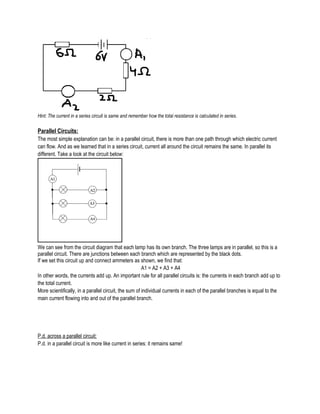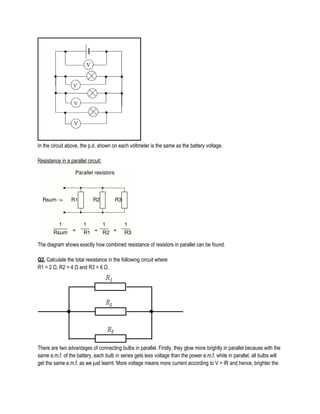Publicité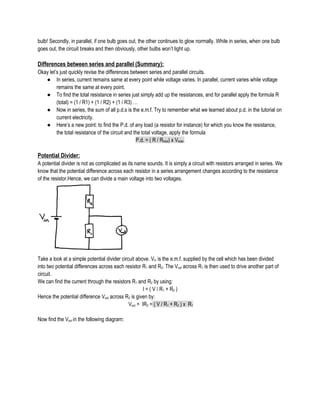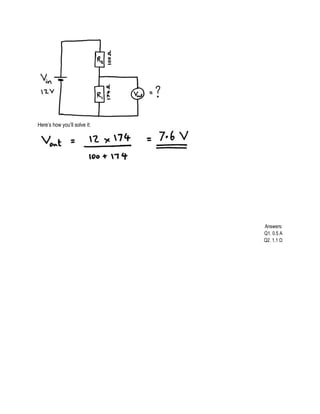Prochain SlideShareSeries & Parallel
Chargement dans ... 3
1 sur 7
Publicité

### D.c.circuits

1. D.C. CIRCUITS! Introduction: Hey again everyone! Hope you all are good. Today we are going to study about D.C. Circuits, D.C. is the abbreviate for direct current. In this tutorial, we’ll study how the arrangement of an electric circuit will affect the electrical properties like charge, current, e.m.f., p.d. and resistance. Ready? Series Circuit: The diagram shows a series circuit. All bulbs and ammeters are connected in series. Do you note that all ammeters are recording the same value? Yeah so here we are: current at any point in a series circuit is the same, no matter how much load you put in. In both bulbs above, same amount of current is flowing. Take a look at the above circuit, it has the same battery and same bulb (only one this time though) and the ammeter is still giving the same reading. Hence, load doesn’t affect the current value in a series circuit. Easy? Let’s move on! Potential Difference across a series circuit:
2. Now in the above diagram, two resistors are connected in series. We know that current will remain same at each point, only the voltage will change. To find the e.m.f. of the battery, simply add up all the voltages in the series circuit, that is: V (e.m.f. of battery) = V1 + V2 So in scientific terms, we can say that in a series circuit, the sum of potential difference across each component is equal to the potential difference across the whole circuit (e.m.f.). Resistance in a series circuit: Coming to the resistance in a series circuit, consider the same circuit. The resistance of each resistor is R1 and R2. We learned the formula V = IR in the tutorial on current electricity. So according to this, p.d. across R1 is V1 is V1 = I R1 and that across R2 is V2 = I R2. We know that V = V1 + V2 V = IR1 + IR2 = I (R1 + R2) Therefore V / I = R1 + R2. From the above derivation we can see that the combined resistance R is the sum of all the resistors in the circuit. Hence in a series circuit, the combined resistance is the sum of all the resistances. Q1. Determine the current in the ammeters A1 and A2.
3. Hint: The current in a series circuit is same and remember how the total resistance is calculated in series. Parallel Circuits: The most simple explanation can be: in a parallel circuit, there is more than one path through which electric current can flow. And as we learned that in a series circuit, current all around the circuit remains the same. In parallel its different. Take a look at the circuit below: We can see from the circuit diagram that each lamp has its own branch. The three lamps are in parallel, so this is a parallel circuit. There are junctions between each branch which are represented by the black dots. If we set this circuit up and connect ammeters as shown, we find that: A1 = A2 + A3 + A4 In other words, the currents add up. An important rule for all parallel circuits is: the currents in each branch add up to the total current. More scientifically, in a parallel circuit, the sum of individual currents in each of the parallel branches is equal to the main current flowing into and out of the parallel branch. P.d. across a parallel circuit: P.d. in a parallel circuit is more like current in series: it remains same!
4. In the circuit above, the p.d. shown on each voltmeter is the same as the battery voltage. Resistance in a parallel circuit: The diagram shows exactly how combined resistance of resistors in parallel can be found. Q2. Calculate the total resistance in the following circuit where R1 = 2 Ω, R2 = 4 Ω and R3 = 6 Ω. There are two advantages of connecting bulbs in parallel. Firstly, they glow more brightly in parallel because with the same e.m.f. of the battery, each bulb in series gets less voltage than the power e.m.f. while in parallel, all bulbs will get the same e.m.f. as we just learnt. More voltage means more current according to V = IR and hence, brighter the
5. bulb! Secondly, in parallel, if one bulb goes out, the other continues to glow normally. While in series, when one bulb goes out, the circuit breaks and then obviously, other bulbs won’t light up. Differences between series and parallel (Summary): Okay let’s just quickly revise the differences between series and parallel circuits. ● In series, current remains same at every point while voltage varies. In parallel, current varies while voltage remains the same at every point. ● To find the total resistance in series just simply add up the resistances, and for parallel apply the formula R (total) = (1 / R1) + (1 / R2) + (1 / R3) … ● Now in series, the sum of all p.d.s is the e.m.f. Try to remember what we learned about p.d. in the tutorial on current electricity. ● Here’s a new point: to find the P.d. of any load (a resistor for instance) for which you know the resistance, the total resistance of the circuit and the total voltage, apply the formula P.d. = ( R / Rtotal) x Vtotal. Potential Divider: A potential divider is not as complicated as its name sounds. It is simply a circuit with resistors arranged in series. We know that the potential difference across each resistor in a series arrangement changes according to the resistance of the resistor.Hence, we can divide a main voltage into two voltages. Take a look at a simple potential divider circuit above. Vin is the e.m.f. supplied by the cell which has been divided into two potential differences across each resistor R1 and R2. The Vout across R1 is then used to drive another part of circuit. We can find the current through the resistors R1 and R2 by using: I = ( V / R1 + R2 ) Hence the potential difference Vout across R2 is given by: Vout = IR2 = ( V / R1 + R2 ) x R1 Now find the Vout in the following diagram:
6. Here’s how you’ll solve it: Answers: Q1. 0.5 A Q2. 1.1 Ω
7. Here’s how you’ll solve it: Answers: Q1. 0.5 A Q2. 1.1 Ω
Publicité